# Norton's Theorem -- Help checking my work please

• Engineering
Homework Statement:
Find V0 from the following circuit using Norton’s theorem only.
Relevant Equations:
Norton's Theorem allowed only
So I've just learned Norton's Theorem and I have this problem on my homework assignment that is wrong. I've checked the answer with a circuit simulator(PSPICE) and the simulation said that V0 should be a drop of 2V. However, my simplified circuit shows a voltage drop of 4V. I have been staring at this problem for an hour trying to find my mistake and I've had no luck. I would be really appreciative if someone could point out where I went wrong.

#### Attachments

• Scan - 2021-03-12 17_45_34.pdf
376.3 KB · Views: 47
•Screen Shot 2021-03-12 at 5.48.07 PM.png
24 KB · Views: 28

## Answers and Replies

It's hard to comment on where your mistake is, except that it's at the beginning. I can't follow your reasoning. Why "∴ IN=2mA"?

BTW, I thought this was a good description:
https://www.allaboutcircuits.com/textbook/direct-current/chpt-10/nortons-theorem/#:~:text=Norton's%20Theorem%20states%20that%20it,resistance%20connected%20to%20a%20load.

That's the description I've been pouring over! But I haven't really had any help from it because the examples they use are so simple! I haven't been able to apply it to the less clear-cut questions.

I just figured that the 12V source with the 12kohm resistor would be 1mA, and it would subtract from the 3mA. Sorry, I should've made that clear. To be honest, I have difficulty figuring out how the electricity travels from its source. Like in this example depending on the path it takes I feel like the sources could either add or subtract. I know this is a flawed understanding, but I would appreciate it if you could tell me why it's flawed.

That being said, I considered the possibility that the currents could add. But if IN was equal to 4mA it would lead to my end voltage source being 16V, which would result in an 8V differential for V0, even further from the answer the simulation gave me!

By the way, thank you for responding! I've been really flustered with this question and the subject in general and I was very worried I would get no responses. I really appreciate you taking the time out of your day to help me! :)

Last edited:
DaveE
Science Advisor
Gold Member
OK, but when you make assumptions at a solution, YOU can check it afterwards to see if it makes sense. This is a key engineering skill because we all make mistakes. Later, when you actually need to use this knowledge, there won't be someone checking your work, or if there is, they won't be impressed with fixing your mistakes. Not being able to solve a problem isn't so bad, in fact, it isn't uncommon in the real world. But thinking you've correctly solved something when you haven't can be a serious problem. That can make bridges collapse, start fires, or cost your employer a lot of money.

I would check your solution for IN like this:

1) Since IN=2mA upward and there is 1mA flowing to the left through the 12V source, then there must be 3mA flowing to the left through the 2KΩ resistor.
2) 3mA in 2KΩ will make a 6V drop. This will make the upper left node -6V since you've shorted the 4KΩ load resistor. The -6V across the leftmost 4KΩ resistor will make 1.5mA flow upwards through it.
3) Now if you sum the currents that are flowing into the bottom node (i.e. downward) you get 3mA - 1.5mA - 2mA - 1mA ≠ 0. So this solution has to be incorrect according to KCL (or conservation of charge, if you prefer).

To do this correctly you must solve for all of the currents on the left side. So, draw current arrows on your schematic to define the polarities then use KCL and ohms law to find the current through ALL of the circuit branches.

Joshy
Gold Member
Okay. It looks like you know the general approach and just need a little nudge.

You got the right idea that you are suppose to solve for short circuit current you called ##I_{N}##. I think the ##I=12V/12k\Omega## came from a mistake probably thinking about the right side of the circuit? Under normal circumstances the voltage above that ##12V## source would not be ##12V## because the voltage would be ##12V## plus the voltage across the resistor that is beneath it. In this case something very happy happens for you when you short it to ground. What is voltage and current when you short the right side to ground?

edit: I made a mistake in my spoiler. It was incorrect. Sorry.
You've shorted it to ground, and so no current is going through that right half circuit. It's like there's nothing there. It's doing nothing and the voltage above and below that ##12V## source is zero. So what you need to do to solve for ##I_{N}## is find the current going through the ##2k\Omega## resistor.

I agree with what you've done for the second part. Something I would recommend not doing is drawing a line with an arrow where ##R_N## is because it'll look like you shorted it while finding the resistance, which increases the risk of making a mistake if you need to rush through the problem in an exam.

Last edited:
•SixOnTheBeach
Okay. It looks like you know the general approach and just need a little nudge.

You got the right idea that you are suppose to solve for short circuit current you called ##I_{N}##. I think the ##I=12V/12k\Omega## came from a mistake probably thinking about the right side of the circuit? Under normal circumstances the voltage above that ##12V## source would not be ##12V## because the voltage would be ##12V## plus the voltage across the resistor that is beneath it. In this case something very happy happens for you when you short it to ground. What is voltage and current when you short the right side to ground?

You've shorted it to ground, and so no current is going through that right half circuit. It's like there's nothing there. It's doing nothing and the voltage above and below that ##12V## source is zero. So what you need to do to solve for ##I_{N}## is find the current going through the ##2k\Omega## resistor.

I agree with what you've done for the second part. Something I would recommend not doing is drawing a line with an arrow where ##R_N## is because it'll look like you shorted it while finding the resistance, which increases the risk of making a mistake if you need to rush through the problem in an exam.

Wow, thank you that's very helpful! I may be misunderstanding you, but is IN 3mA, as the only thing pushing current through is the 3mA source? Why does shorting the resistor make the 12V source zero? Can the voltage from it not still travel through the 2k resistor?

Joshy
Gold Member
I made a mistake. I'm sorry.

edit:

I'm going to try this myself to verify. Apologies.

edit 2:

I made a mistake when I told you that the right side had no current going through. That was wrong. This was a good question. What makes this tricky is you have to think of the short circuit drawing as like two separate circuits.

An ideal wire is going to have no voltage drop across it and so if it's connected to ground, then the voltage there is zero. The right half circuit the total voltage across it is zero, but it doesn't mean there's no current going through it. To "cancel" out the ##12V## source there's current going through the resistor beneath it so that the voltage across it is ##-12V##. You can use Ohm's law to get its current.

Since there is a short... none of the current from that right side of the circuit wants to go the left half. That's because current travels towards the least resistive path.

The left half of the circuit looks like you're already familiar with it. That's a current divider. You know that the ##3mA## is going to have to split between those two resistors. You want the current going through the ##2k\Omega## resistor because that's the one connected to ##I_N##.

Since there is a short... none of the current from the left side of the circuit wants to go the right half.

This means that the current going through the short is going to be both currents (the current from the left, and the current from the right). If you sum it together you'll get the correct ##I_N##.

Last edited:
•SixOnTheBeach
This problem requires that we only use Norton's theorem to solve it, so it seems that we can't even use KVL and KCL, but it does not limit the number of times we use Norton's theorem.•SixOnTheBeach and DaveE
DaveE
Science Advisor
Gold Member
This problem requires that we only use Norton's theorem to solve it, so it seems that we can't even use KVL and KCL, but it does not limit the number of times we use Norton's theorem.It's an odd requirement though, pedantically speaking. You are left solving a current divider on the left side, which I claim is impossible to do without Kirchhoff laws or Thevenin's (source transformation). Even two series resistors is simplified with KVL. Granted, it is the simplest possible example of those laws, but still...

Last edited:
•SixOnTheBeach
DaveE
Science Advisor
Gold Member
I think you should follow up with @Joshy 's advice to split the problem into two parts, left side and right side. Because you have replaced Ro with a short circuit, you can just add those two currents together (the current from the left and from the right). There is no voltage generated in the short circuit branch. so there is no interaction, i.e. a change in the current from one side has no effect on the circuit on the other side.

The next step is to solved the Norton equivalent for the left side. You've already done the right side correctly. Then you can combine the two and rearrange to end up with one current source and one parallel resistor for the final configuration. This is much clearer if you redraw the circuit for each step of the manipulation. as I've sketched out below.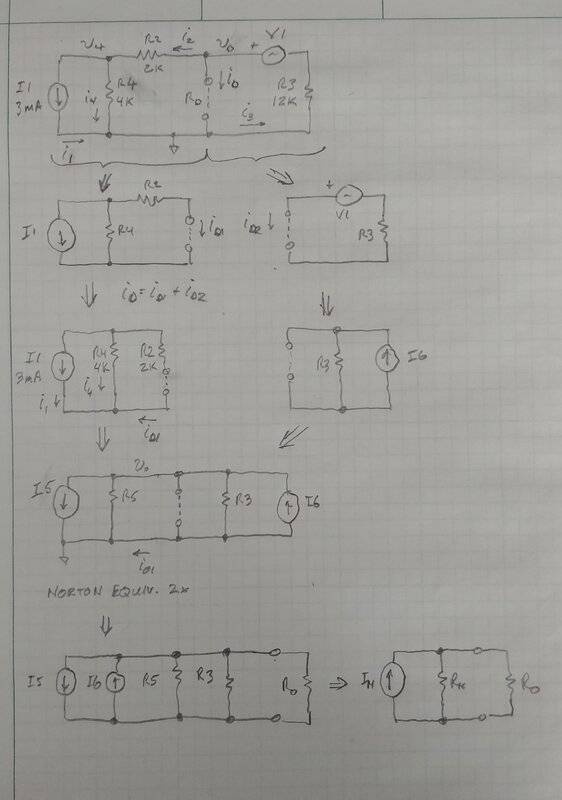•alan123hk and SixOnTheBeach
This problem requires that we only use Norton's theorem to solve it, so it seems that we can't even use KVL and KCL, but it does not limit the number of times we use Norton's theorem.I could be wrong on this, but I believe KVL and KCL are allowed! I think he meant moreso you can't use source transformations, thevenins, etc, basically anything above the very basics.

•alan123hk
I think you should follow up with @Joshy 's advice to split the problem into two parts, left side and right side. Because you have replaced Ro with a short circuit, you can just add those two currents together (the current from the left and from the right). There is no voltage generated in the short circuit branch. so there is no interaction, i.e. a change in the current from one side has no effect on the circuit on the other side.

The next step is to solved the Norton equivalent for the left side. You've already done the right side correctly. Then you can combine the two and rearrange to end up with one current source and one parallel resistor for the final configuration. This is much clearer if you redraw the circuit for each step of the manipulation. as I've sketched out below.
View attachment 279722
Thank you so much! This was VERY helpful. I totally understand why R3 would be in parallel. I followed this methodology and arrived at 1.85V drop for the 4kΩ, which still varies from the 2V I found in the simulation, but very close! This could possibly just be due to internal errors. I arrived at R2 || R3 || R4 equaling resistance of 1.2kΩ, and going off of the work you showed because I5 and I6 are in parallel then I am correct in thinking that IN should be 2mA. So with a 2mA source in parallel with the 1.2kΩ resistor and the 4kΩ load resistor in our Norton's circuit, it can be reduced to a 2.4V source in series with these resistors. This results in an overall current of 0.46mA which multiplied by the 4kΩ resistor results in the 1.85V drop. Is this logic all correct?

Joshy
Gold Member
No no. You can get the exact answer. Check out what Dave drew in #`10 that can help break it down to two easier problems. Can you post your work so that maybe we can point out what may have gone wrong?

Since this isn't solving the problem I wanted to clarify that the voltage above that resistor is ##-2V##.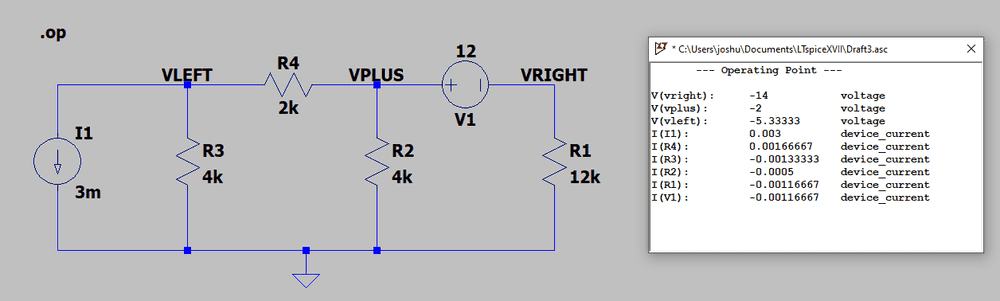You can get the exact answer by hand no problem.

Do the two separate circuits like Dave drew. Get the currents in both circuits. Tell us what you get.

edit:

I think the mistake I am hearing is the equivalent resistance part now. Not all of the resistors are parallel when you solve for ##R_{N}##.

DaveE
Science Advisor
Gold Member
very close!
Close doesn't really count for this sort of problem. Some mistakes make big errors, others make small errors. The size of the error isn't necessarily related to the significance of the mistake. The correct answer is 2V.

I arrived at R2 || R3 || R4 equaling resistance of 1.2kΩ
Mathematically correct, but not very useful. R2 is NOT in parallel with R3. This is obvious from the schematics (all of them).

because I5 and I6 are in parallel then I am correct in thinking that IN should be 2mA.
No. I can't tell what your reasoning is here, but it's wrong. Why do you think I created new labels for some of the resistors and current sources? Why do you assume I5 = I1? The circuit surrounding it has changed. Similar to the way we changed V1 into I6. This is the point with these equivalent circuits: the circuits are equivalent, but that doesn't mean all of the values remain the same.

I think you are still operating in the mode of guessing at the difficult steps finishing the problem and asking if you were right. This is not a good approach to understanding. Otherwise you could have stopped at the computer simulation. "The computer says the answer is 2V, but I don't know why" won't teach you much about circuits.

What I think you need to focus on is using the Norton equivalence process (finding the short circuit current and then the impedance seen by the load). Once on the right side, and again on the left side. This boils down to determining the values for the components that I labeled R5, I5, and I6 by following the process shown in the web link I posted.

Hint: You must solve the problem of how much current flows through R2 when the load Ro is shorted. Focus on the last schematic in the progression I posted that still has R2 in it. That circuit (2 resistors in parallel with a current source) is about as easy as I can make it.

DaveE
Science Advisor
Gold Member
I could be wrong on this, but I believe KVL and KCL are allowed! I think he meant moreso you can't use source transformations, thevenins, etc, basically anything above the very basics.
Which is too bad, since that's the easier way, at least for me.

I could be wrong on this, but I believe KVL and KCL are allowed! I think he meant moreso you can't use source transformations, thevenins, etc, basically anything above the very basics.

I think you also make sense.It's an odd requirement though, pedantically speaking. You are left solving a current divider on the left side, which I claim is impossible to do without Kirchhoff laws or Thevenin's (source transformation). Even two series resistors is simplified with KVL. Granted, it is the simplest possible example of those laws, but still...

I agree with you.For the circuit on the left, we need to use Kirchhoff's law or Thevenin's theorem to determine the short-circuit current to construct the Norton equivalent circuit.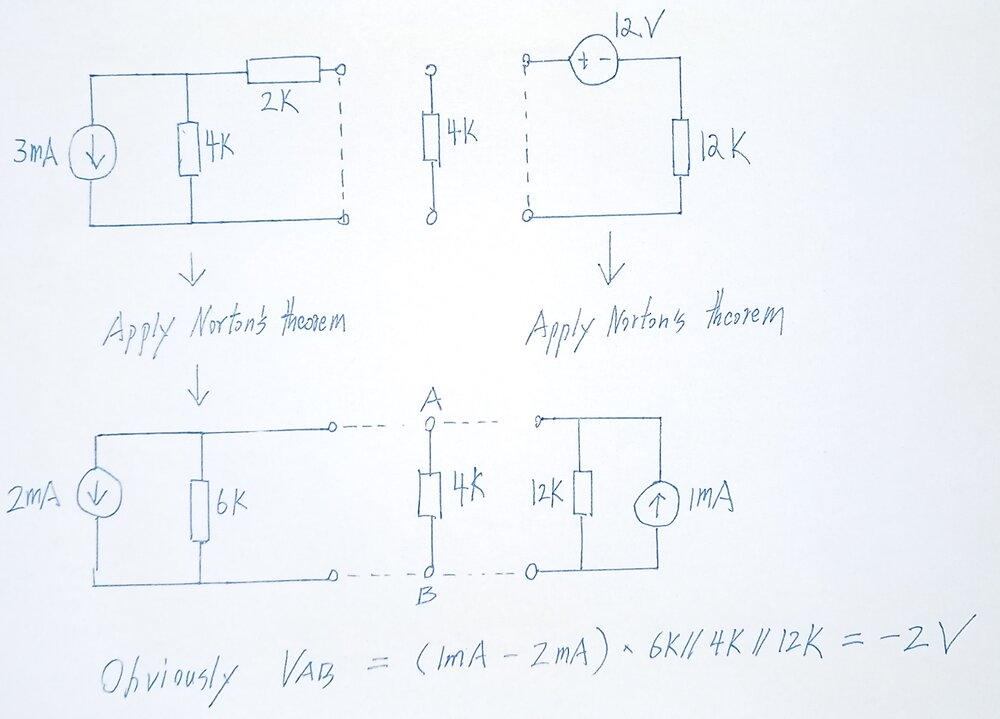Joshy
Gold Member
May you please solve these two circuits and get the current ##I_1## and ##I_2##? Showing your work is a good idea :)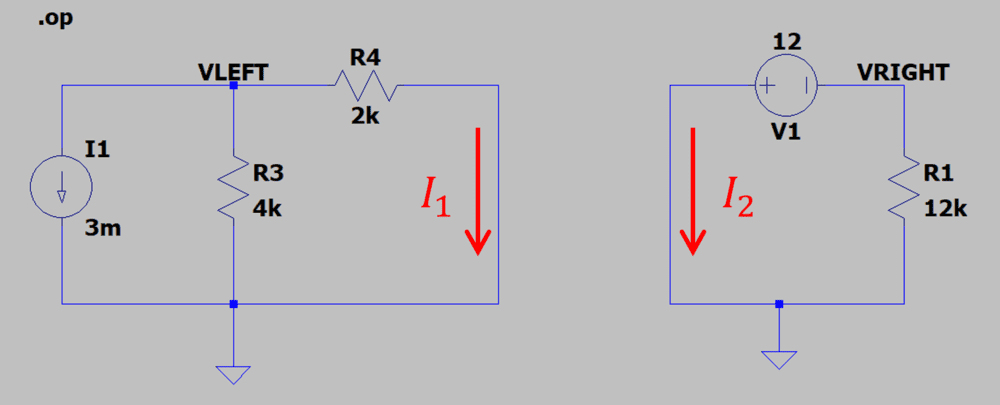Then solve for ##R_{IN}##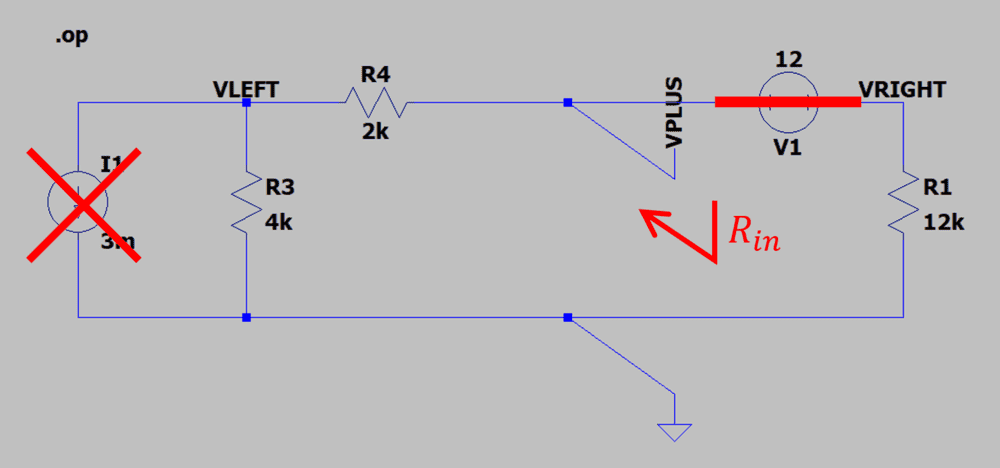I think you also make sense.I agree with you.For the circuit on the left, we need to use Kirchhoff's law or Thevenin's theorem to determine the short-circuit current to construct the Norton equivalent circuit.

View attachment 279730
Ah, I finally see my mistake! Since the 3mA source was in parallel with the 4kΩ resistor it can be thought of as a 12V source. However, when you bring in the 2kΩ resistor, the 12V voltage will mean a 2mA current (12/6 instead of 12/4). When I use a 2mA current source in my work, it leads to a final source of 1mA instead of 2mA which halves my answer leading to the correct answer. Thank you so much, everyone! It kinda warms my heart so many people would spend this much time helping me out at no gain to themselves <3

EDIT: Maybe I'm wrong but I believe you made a small mistake at the end? From what I have and from what everyone has shown me, I believe at the end it should just be 6kΩ || 12kΩ without the 4kΩ resistor as that's the load resistor. I did the math and having all 3 in parallel lead me to a resistance of ~13kΩ, which would not equal 2V when multiplied by -1mA. However, if you create the Norton's circuit with the 4kΩ resistor(from 6kΩ || 12kΩ) in combination with the 4kΩ load resistor, they will be in series once the current is transformed to a voltage source(leading to a total resistance of 8kΩ, not 13kΩ). I'm sorry if my explanation was confusing, I'm putting my work underneath:

#### Attachments

• Scan - 2021-03-18 12_23_10.pdf
950.7 KB · Views: 20
Last edited:
DaveE
Science Advisor
Gold Member
Ah, I finally see my mistake! Since the 3mA source was in parallel with the 4kΩ resistor it can be thought of as a 12V source. However, when you bring in the 2kΩ resistor, the 12V voltage will mean a 2mA current (12/6 instead of 12/4). When I use a 2mA current source in my work, it leads to a final source of 1mA instead of 2mA which halves my answer leading to the correct answer. Thank you so much, everyone! It kinda warms my heart so many people would spend this much time helping me out at no gain to themselves <3
Yes, good work.

I did the math and having all 3 in parallel lead me to a resistance of ~13kΩ
What? Very confusing. In a circuit with resistances of 2K, 6K, 4K, 12K... all <13K, there is no way that you can calculate a parallel combination of 13K, which is larger than any of the individual resistors. You do not ever increase the resistance in a circuit by adding a resistor in parallel. You didn't show us your math, but I am 99.99999% sure it's wrong.

Anyway, it doesn't matter. Since now you know the correct way to analyze this circuit.

•SixOnTheBeach